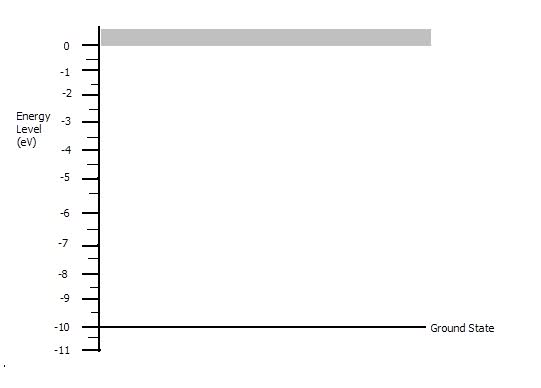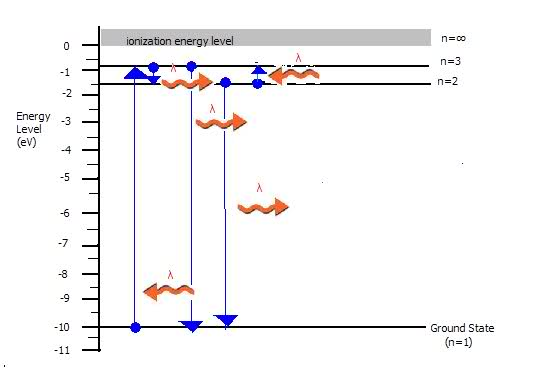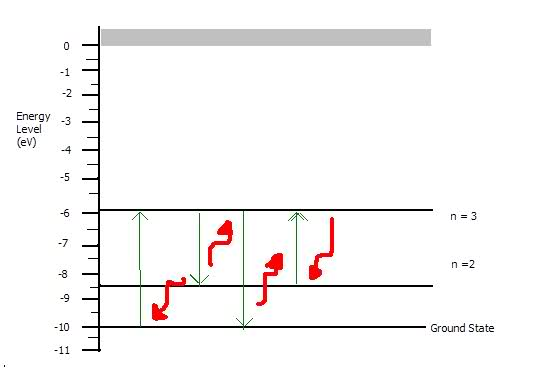# Energy Level Diagram Check

1. Problem:
The ground-state energy of a hypothetical atom is at -10.0eV. When these atoms, in the ground state, are illuminated with light, only the wavelengths of 207 nanometers and 146 nanometers are absorbed by the light atoms (1 nanometer = 10^-9meters).

(a)Calculate the energies of the photons of light of the two absorption-spectrum wavelengths.

(b)Complete the energy level diagram shown below for these atoms by showing all the excited energy states.(c)Show by arrows on the energy-level diagram all of the possible transitions that would produce emission-spectrum lines.

(d)What would be the wavelength of the emission line corresponding to the transition from the second excited state to the first excited state?

## Homework Equations

E=13.6 [(1/ni^2)-(1/n2^2)]eV

En=-(13.6eV)[(Z^2)/(n^2)]

(1/λ)=(2pi^2mk^2e^4/h^3c)[(1/nf^2)-(1/ni^2)]

2pi^2mk^2e^4/h^3c = 1.097 x 10^7 m

E = hc/λ

hc=1.24 x 10^3eV

## The Attempt at a Solution

(a)
207nm = 2.07 x 10^-7m
146nm = 1.46 x 10^-7m
E = hc/λ
E1=[(1.24 x 10^3eV)/(2.07 x 10^-7)]
E1=5.99 x 10^9

E2=[(1.24 x 10^3eV)/1.46 x 10^-7)]
E2=8.493 x 10^9

(b)
I assumed it was bohr model...(?)
Ground state is at -10.0eV
First excited state is 1/4 of ground state
=(1/4)(-10.0eV)
first excited state=-2.5eV
Second excited state is 1/9 of ground state
=(1/9)(-10.0eV)
second excited state is -1.11eV(c) is included in picture (?) squiggly arrows..

(d)
ni=2
nf=1

(1/λ)=(2pi^2mk^2e^4/h^3c)[(1/nf^2)-(1/ni^2)]
(1/λ)=(1.097 x 10^7 m)[(1/(1)^2)-(1/(2)^2)]
(1/λ)=(1.097 x 10^7 m)[(1/1)-(1/4)]
(1/λ)=(1.097 x 10^7 m)(1-0.25)
(1/λ)=(1.097 x 10^7 m)(0.75)
(1/λ)=8.2275 x 10^6
1=(8.2275 x 10^6)λ
λ=(1/8.2275 x 10^6)
λ=1.2154 x 10^-7m

Redbelly98
Staff Emeritus
Homework Helper

## Homework Equations

E=13.6 [(1/ni^2)-(1/n2^2)]eV

En=-(13.6eV)[(Z^2)/(n^2)]

(1/λ)=(2pi^2mk^2e^4/h^3c)[(1/nf^2)-(1/ni^2)]

2pi^2mk^2e^4/h^3c = 1.097 x 10^7 m

E = hc/λ

hc=1.24 x 10^3eV
Right number, wrong units. It's actually 1.24 x 103 eV·nm

## The Attempt at a Solution

(a)
207nm = 2.07 x 10^-7m
146nm = 1.46 x 10^-7m
E = hc/λ
E1=[(1.24 x 10^3eV)/(2.07 x 10^-7)]
E1=5.99 x 10^9

E2=[(1.24 x 10^3eV)/1.46 x 10^-7)]
E2=8.493 x 10^9
Not quite. Try calculating E1 and E2 again, using the correct units for hc (eV·nm) and λ (nm).

(b)
I assumed it was bohr model...(?)
Not a bad idea, but then the absorbed wavelengths would turn out to be different than the ones they said. So it turns out this one does not follow the Bohr model.

Instead, you can use E1 and E2 to figure out where the energy levels are.

So to try this again.

For part a)
E1 from 207nm lambda comes out to be 5.99 and E2 from 146nm to be 8.4935

For part b)
You draw those two levels as excited states on the diagramThen for part d)
I keep thinking of using the same (1/lambda) equation to find the wavelength with nf being 2 and ni being 1 but since this isn't a bohr model, that equation doesn't apply..(?)

Meaning... I would have to use I would have to use E1 and E2 ...
Find the energy w/i E1 and E2 by taking E2 - E1
-8.49-(-5.99)
giving me DeltaE = -2.503eV

Then setting that total e and rearranging E=(hc/lambda) to find lambda giving me
Lambda = (hc/E)
Lambda = [(1.24 x 10^3eV*nm)/(-2.503eV)]
Which gives the final answer lambda = -495.406... 495.406nm wavelength of emission??

Correct? Maybe not?

Redbelly98
Staff Emeritus
Homework Helper
So to try this again.

For part a)
E1 from 207nm lambda comes out to be 5.99 and E2 from 146nm to be 8.4935

You're getting there!

Those are the correct energies of the photons.

If a ground state atom absorbs a 207 nm photon, it's energy will be 5.99 eV higher than it was in the ground state. So, what would be the energy level after absorbing a 207 nm photon?

a) Calculated E1 = 5.99eV and E2 = 8.49eV as energies given off
b) From ground state, -10.0eV + 5.99eV gives me -4.01eV
-10.0eV + 8.49315 gives me = -1.50685eV
Meaning on my diagram, the first excited state would be -4.01eV and second being -1.50685
d) using lambda = (hc/E)
I first find the energy difference from n=2 to n=1
-1.506 - (-4.01)
change in E = 2.5035eV

Then substituting lambda = [(1.24 x 10^3eV*nm)/2.5035eV)]
lambda = 495.307m

Redbelly98
Staff Emeritus
Looks good